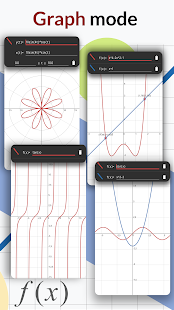A scientific calculator supports most of the features of 500 ms 500 es 570 ms 570 es 991 es 991 ms and 82 ms & 82 es. Calculator’s most advanced scientific calculator to date, has been designed and engineered for easy operation at any level.

Modern theme

A scientific calculator supports most of the features of 500 ms 500 es 570 ms 570 es 991 es 991 ms and 82 ms & 82 es. Calculator’s most advanced scientific calculator to date, has been designed and engineered for easy operation at any level. The Natural Display and enhanced features like displaying root forms of equations, finding derivatives, product notation templates, and advanced inequality functionality, makes the fx calculator plus extremely versatile and the perfect choice for high school and college students alike. This is all in one calculator, support full mathematics function (more than 100 functions) and working offline.

All General Mathematics courses
– Pre-Algebra
– Algebra
– Statistics
– Pre-Calculus
– Trignonmetry
– Chemistry
– Physics
– Engineering

Functionality
– Natural textbook display shows expressions and results exactly as they appear in the textbook
– Over 280 functions, including fractions, statistics, complex number calculations, base arithmetic, derivative, integral, linear regression, standard deviation, computer science, and polar-rectangular conversions
– List-Based STAT Data Editor
– Table Function
– Matrix and Vector Calculations
– 40 Metric Conversions
– Multi-replay function allows you to backtrack step-by-step through calculations, where you can edit expressions and recalculate answers

Screenshots

•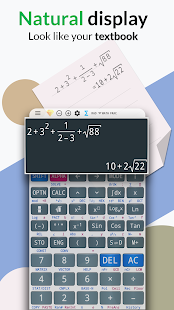•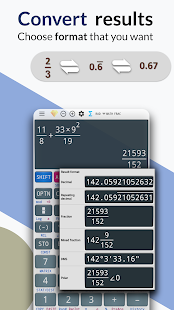••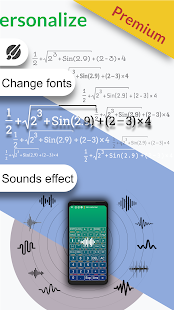•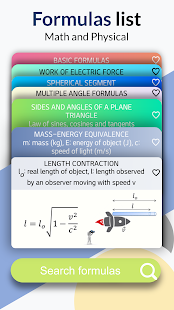•